# Introduction

In the last note, we introduced the concept of isolation as one of the ACID properties. Let’s revisit our definition here:

• Isolation: Execution of each Xact is isolated from that of others. In reality, the DBMS will interleave actions of many Xacts and not execute each in order of one after the other. The DBMS will ensure that each Xact executes as if it ran by itself.

This note will go into details on how the DBMS is able to interleave the actions of many transactions, while guaranteeing isolation.

# Two Phase Locking

What are locks, and why are they useful? Locks are basically what allows a transaction to read and write data. For example, if Transaction $$T1$$ is reading data from resource $$A$$, then it needs to make sure no other transaction is modifying resource $$A$$ at the same time. So a transaction that wants to read data will ask for a Shared (S) lock on the appropriate resource, and a transaction that wants to write data will ask for an Exclusive (X) lock on the appropriate resource. Only one transaction may hold an exclusive lock on a resource, but many transactions can hold a shared lock on data. Two phase locking (2PL) is a scheme that ensures the database uses conflict serializable schedules. The two rules for 2PL are:

• Transactions must acquire a S (shared) lock before reading, and an X (exclusive) lock before writing.

• Transactions cannot acquire new locks after releasing any locks – this is the key to enforcing serializability through locking!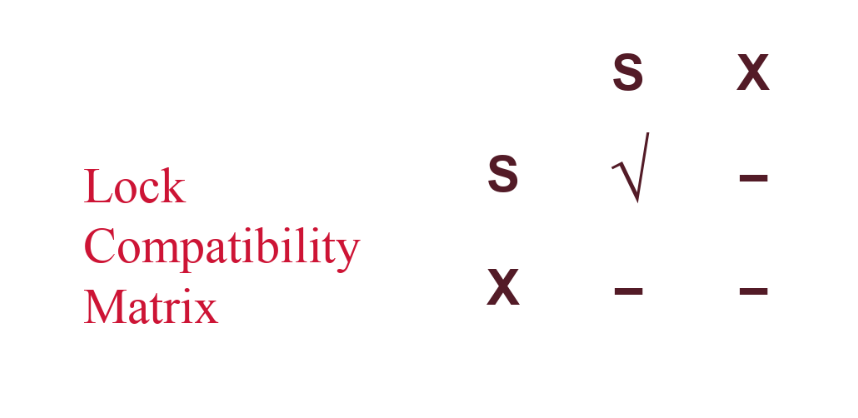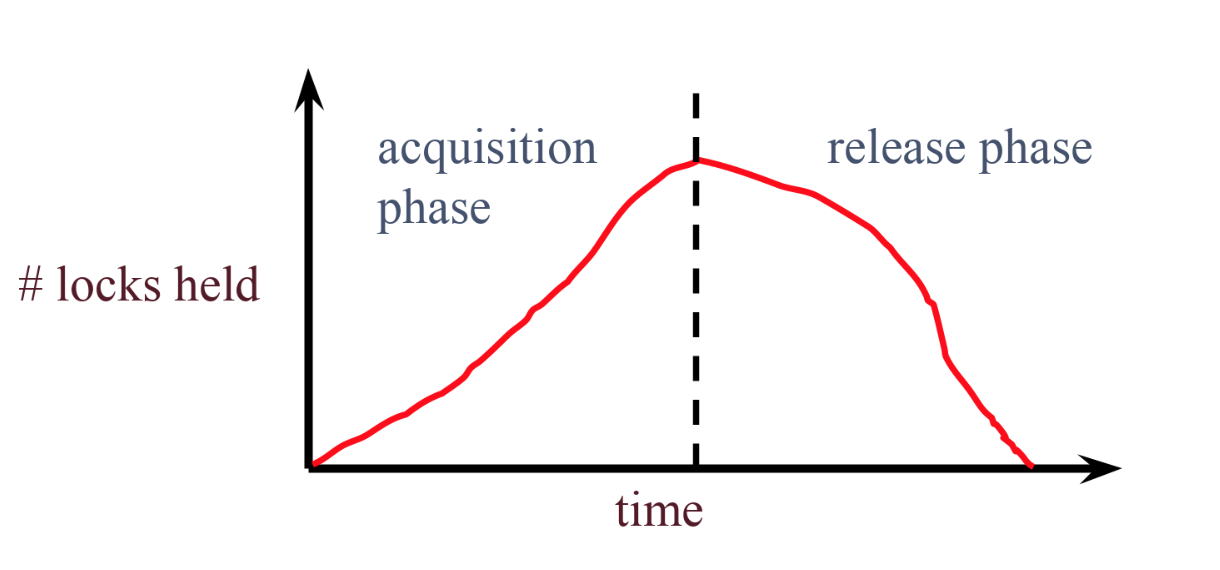Two Phase Locking guarantees conflict serializability. When a committing transaction has reached the end of its acquisition phase, lets call this the “lock point”. At this point, it has everything that it needs locked. Any conflicting transactions either started the release phase before this point or are blocked waiting for this transaction. So the visibility of actions of two conflicting transactions can be ordered by their lock points. The order of these lock points gives us an equivalent serial schedule!

The problem with this is that it does not prevent cascading aborts. For example,

• $$T1$$ updates resource $$A$$ and then releases the lock on $$A$$.

• $$T2$$ reads from $$A$$.

• $$T1$$ aborts.

• In this case, $$T2$$ must also abort because it read an uncommitted value of $$A$$.

To solve this, we will use Strict Two Phase Locking. Strict 2PL is the same as 2PL, except all locks get released together when the transaction completes.# Lock Management

Now we know what locks are used for and the types of locks. We will take a look at how the Lock Manager1 manages these lock and unlock (or acquire and release) requests and how it decides when to grant the lock. The LM maintains a hash table, keyed on names of the resources being locked. Each entry contains a granted set (a set of granted locks/the transactions holding the locks for each resource), lock type (S or X or types we haven’t yet introduced), and a wait queue (queue of lock requests that cannot yet be satisfied because they conflict with the locks that have already been granted). See the following graphic: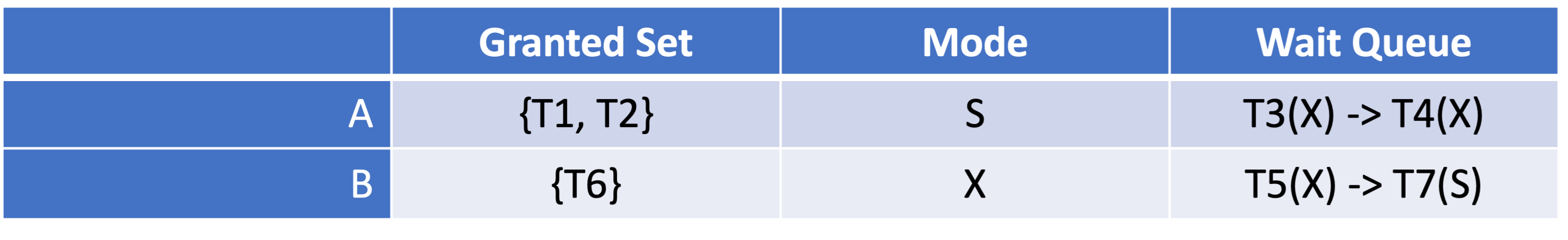When a lock request arrives, the Lock Manager checks if any Xact in the Granted Set or in the Wait Queue want a conflicting lock. If so, the requester gets put into the Wait Queue. If not, then the requester is granted the lock and put into the Granted Set. In addition, Xacts can request a lock upgrade: this is when a Xact with shared lock can request to upgrade to exclusive. The Lock Manager will add this upgrade request at the front of the queue.

Here is some pseudocode for how to process the queue; note that it doesn’t explicitly go over what to do in cases of promotion etc, but it’s a good overview nevertheless.

# If queue skipping is not allowed, here is how to process the queue

H = set of held locks on A
Q = queue of lock requests for A

def request(lock_request):
if Q is empty and lock_request is compatible with all locks in H:
grant(lock_request)
else:

def release_procedure(lock_to_release):
release(lock_to_release)
for lock_request in Q:        # iterate through the lock requests in order
if lock_request is compatible with all locks in H:
grant(lock_request)   # grant the lock, updating the held set
else:
return


Note that this implementation does not allow queue skipping. When a request arrives under a queue skipping implementation, we first check if you can grant the lock based on what locks are held on the resource; if the lock cannot be granted, then put it at the back of the queue. When a lock is released and the queue is processed, grant any locks that are compatible with what is currently held.

For an example of queue skipping and pseudocode, see the appendix. It relies on you understanding multigranulariy locking however, so make sure to read section 7 first to understand the example.

We now have a lock manager that will put requesters into the Wait Queue if there are conflicting locks. But what happens if $$T1$$ and $$T2$$ both hold $$S$$ locks on a resource and they both try upgrade to $$X$$? $$T1$$ will wait for $$T2$$ to release the $$S$$ lock so that it can get an $$X$$ lock while $$T2$$ will wait for $$T1$$ to release the $$S$$ it can get an $$X$$ lock. At this point, neither transaction will be able to get the $$X$$ lock because they’re waiting on each other! This is called a deadlock, a cycle of Xacts waiting for locks to be released by each other.

## Avoidance

One way we can get around deadlocks is by trying to avoid getting into a deadlock. We will assign the Xact’s priority by its age: now - start time. If $$Ti$$ wants a lock that $$Tj$$ holds, we have two options:2

• Wait-Die: If $$Ti$$ has higher priority, $$Ti$$ waits for $$Tj$$; else $$Ti$$ aborts

• Wound-Wait: If $$Ti$$ has higher priority, $$Tj$$ aborts; else $$Ti$$ waits Please read the diagram below like a ternary operator (C/C++/java/javascript)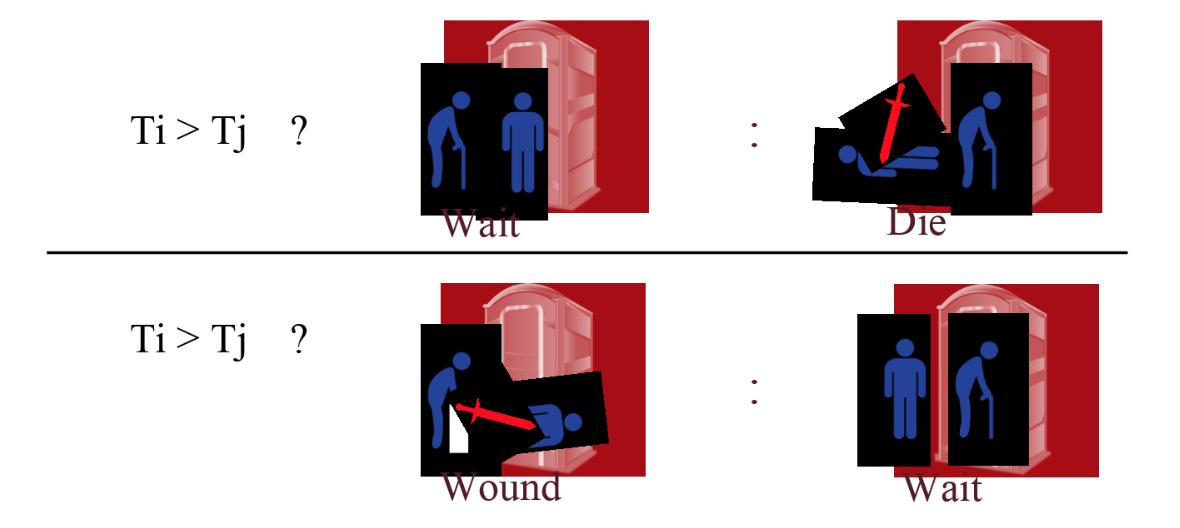## Detection

Although we avoid deadlocks in the method above, we end up aborting many transactions! We can instead try detecting deadlocks and then if we find a deadlock, we abort one of the transactions in the deadlock so the other transactions can continue. We will detect deadlocks by creating and maintaining a “waits-for” graph. This graph will have one node per Xact and an edge from $$T_i$$ to $$T_j$$ if:

• $$T_j$$ holds a lock on resource X

• $$T_i$$ tries to acquire a lock on resource X, but $$T_j$$ must release its lock on resource X before $$T_i$$ can acquire its desired lock.

For example, the following graph has a edge from $$T1$$ to $$T2$$ because after $$T2$$ acquires a lock on B, $$T1$$ tries to acquire a conflicting lock on it. Thus, $$T1$$ waits for $$T2$$.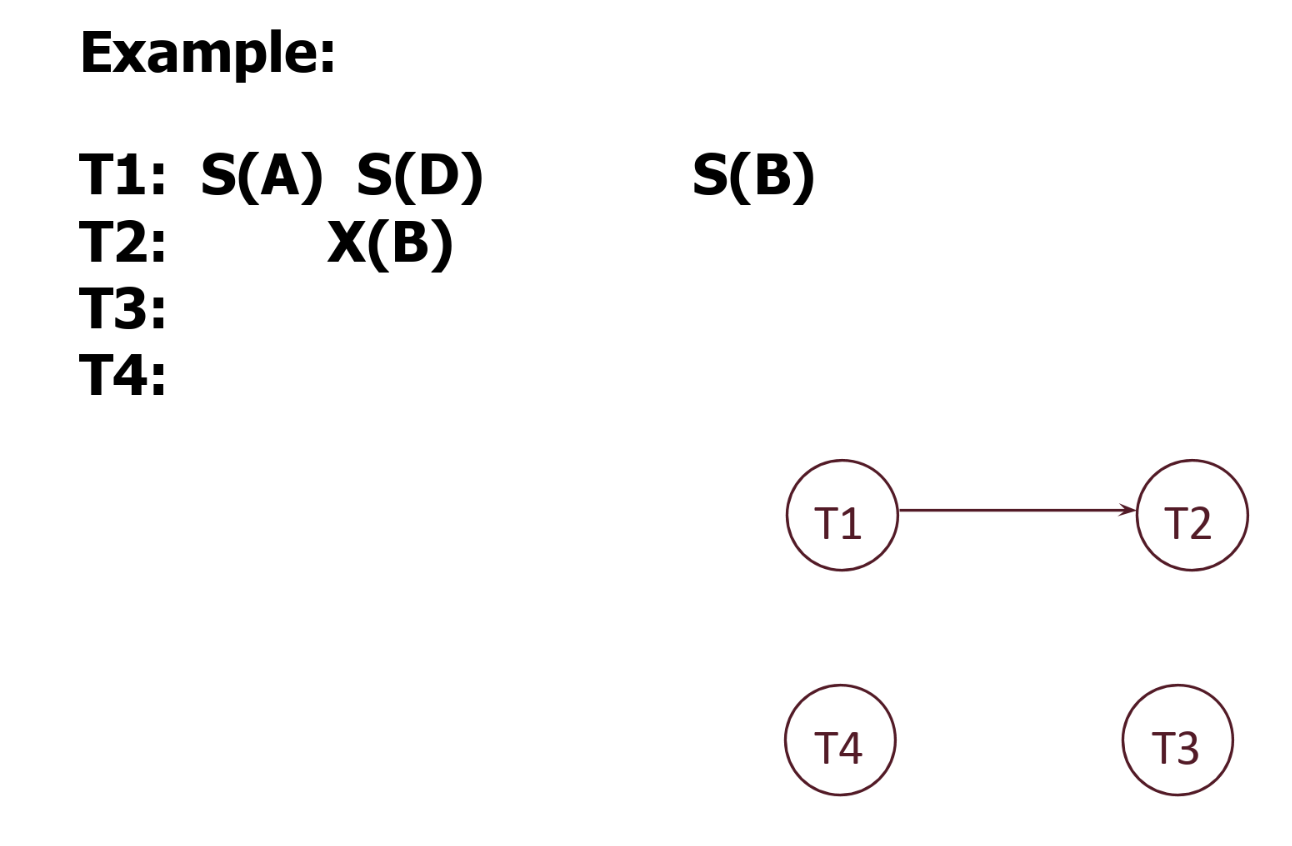If a transaction $$T_i$$ is waiting on another transaction $$T_j$$ (i.e. there is an edge from $$T_i$$ to $$T_j$$), then $$T_i$$ cannot acquire any new locks. Therefore, a transaction $$T_k$$ will not wait for $$T_i$$ on a resource $$X$$ unless $$T_i$$ had acquired a conflicting lock on $$X$$ before it began waiting for $$T_j$$.
Consider the example below, while keeping in mind that only lock acquisitions are shown in schedule, not lock releases.\There is an edge from $$T2$$ to $$T1$$ because $$T1$$ holds an X lock, when $$T2$$ requests a conflicting S lock on resource A. Once $$T2$$ waits for $$T1$$ to finish with resource A, none of $$T2$$’s operations can proceed until it is removed from the wait queue. This is why $$T3$$ does not wait for $$T2$$ when acquiring an S lock on B, since $$T2$$ was never actually able to acquire an X lock on B, as it was still waiting on $$T1$$. Similarly, when $$T3$$ goes to acquire an X lock on A, it need only wait for $$T1$$ since at that point in time the only transaction with a conflicting lock on A is $$T1$$. Note that at that point both $$T2$$ and $$T3$$ will be in the wait queue for resource A. We will periodically check for cycles in a graph, which indicate a deadlock. If a cycle is found, we will "shoot" a Xact in the cycle and abort it to break the cycle. An interesting empirical fact is that most deadlock cycles are small (2-3 transactions). Important note: A "waits-for" graph is used for cycle detection and is different from the conflict dependency graph we discussed earlier (in the previous note) which was used to figure out if a transaction schedule was serializable. As a reminder: Conflict Dependency Graph: Draw an edge from Ti to Tj iff

• Tj and Ti operate on the same resource, with Ti operation preceding Tj op.

• At least one of Ti and Tj is a write.

• Used to determine conflict serializability

Waits-For Graph: Draw an edge from Ti to Tj iff

• Tj holds a conflicting lock on the resource Ti wants to operate on, meaning Ti must wait for Tj

# Lock Granularity

So now that we understand the concept of locking, we want to figure out what to actually lock. Do we want to lock the tuple containing the data we wish to write? Or the page? Or the table? Or maybe even the entire database, so that no transaction can write to this database while we’re working on it? As you can guess, the decision we make will differ greatly based upon the situation we find ourselves in. Let us think of the database system as the tree below: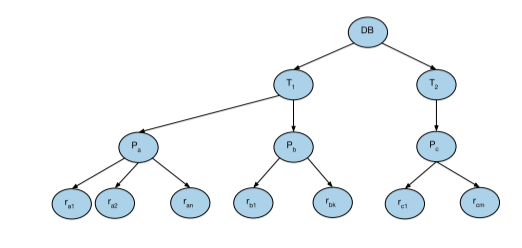The top level is the database. The next level is the table, which is followed by the pages of the table. Finally, the records of the table themselves are the lowest level in the tree. Remember that when we place a lock on a node, we implicitly lock all of its children as well (intuitively, think of it like this: if you place a lock on a page, then you’re implicitly placing a lock on all the records and preventing anyone else from modifying it). So you can see how we’d like to be able to specify to the database system exactly which level we’d really like to place the lock on. That’s why multigranularity locking is important; it allows us to place locks at different levels of the tree. We will have the following new lock modes:

• IS: Intent to get S lock(s) at finer granularity.

• IX: Intent to get X lock(s) at finer granularity. Note: that two transactions can place an IX lock on the same resource – they do not directly conflict at that point because they could place the X lock on two different children! So we leave it up to the database manager to ensure that they don’t place X locks on the same node later on while allowing two IX locks on the same resource.

• SIX: Like S and IX at the same time. This is useful if we want to prevent any other transaction from modifying a lower resource but want to allow them to read a lower level. Here, we say that at this level, I claim a shared lock; now, no other transaction can claim an exclusive lock on anything in this sub-tree (however, it can possibly claim a shared lock on something that is not being modified by this transaction–i.e something we won’t place the X lock on. That’s left for the database system to handle).

Interestingly, note that no other transaction can claim an S lock on the node that has a SIX lock, because that would place a shared lock on the entire tree by two transactions, and that would prevent us from modifying anything in this sub-tree. The only lock compatible with SIX is IS. Here is the compatibility matrix below; interpret the axes as being transaction $$T1$$ and transaction $$T2$$. As an example, consider the entry X, S – this means that it is not possible for $$T1$$ to hold an X lock on a resource while $$T2$$ holds an S lock on the same resource. NL stands for no lock.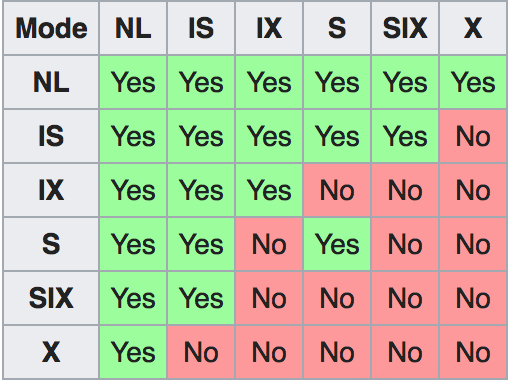## Multiple Granularity Locking Protocol

1. Each Xact starts from the root of the hierarchy.

2. To get S or IS lock on a node, must hold IS or IX on parent node.

3. To get X or IX on a node, must hold IX or SIX on parent node.

4. Must release locks in bottom-up order.

5. 2-phase and lock compatibility matrix rules enforced as well

6. Protocol is correct in that it is equivalent to directly setting locks at leaf levels of the hierarchy.

# Practice Problems

1. Is the following schedule possible under 2PL? S means acquiring a shared lock, X means acquiring an exclusive lock, and U means releasing a lock.2. Is the above schedule possible under strict 2PL?

3. For the schedule below, which (if any) transactions will wait under a "wait-die" deadlock avoidance strategy? The priorities in descending order are: T1, T2, T3, T4.34. For the schedule above, which (if any) transactions will wait under a "wound-wait" deadlock avoidance strategy? The priorities in descending order are: T1, T2, T3, T4.

5. What does the "waits-for" graph look like for the above schedule from problem 3? Is there deadlock?

6. For the database system below, which lock modes (including IS, IX, or SIX) on which resources are necessary to read $$P_a$$?7. For the database system above, which lock modes (including IS, IX, or SIX) held by other transactions on $$P_a$$ would prevent us from modifying $$r_{a1}$$?

# Solutions

1. Yes, the schedule is possible under 2PL, because no transaction acquires a lock after it begins to release locks.

2. No, the schedule is not possible under strict 2PL, because $$T1$$ does not release all of its locks at once. Instead, $$T3$$ is able to acquire a lock on A after $$T1$$ releases the X lock on A, but before $$T1$$ releases the X lock on C. Therefore, the schedule violates strict 2PL since $$T3$$ could potentially abort under a cascading abort.

3. $$T1$$ and $$T3$$
TS refers to timestep (the top row in the schedule).
$$T2$$ will abort at TS-2 since $$T2$$ has lower priority than $$T1$$. $$T3$$ will wait for $$T4$$ at TS-5 since $$T3$$ has higher priority than $$T4$$. $$T1$$ will wait for $$T4$$ at TS-7 since $$T1$$ has higher priority than $$T4$$.

4. $$T2$$
$$T2$$ will wait for $$T1$$ at TS-2 since $$T2$$ has lower priority than $$T1$$. $$T4$$ will abort at TS-5 since $$T3$$ has higher priority than $$T4$$.

5. There is no deadlock, because there is no cycle in the waits-for graph.There is an edge from $$T2$$ to $$T1$$ since $$T2$$ waits for $$T1$$ at TS-2. This means there is no edge from $$T2$$ to $$T4$$ at TS-4 since $$T2$$ is already waiting for another transaction. There is an edge from $$T3$$ to $$T4$$ at TS-5. There is also an edge from $$T1$$ to $$T4$$ at TS-7.

6. We would need the IS lock mode on $$DB$$ and $$T_1$$, and the S lock mode on $$P_a$$. This allows us to read from $$P_a$$ while restricting other transactions as little as possible.

7. S, SIX, and X lock modes held by other transactions on $$P_a$$ would prevent us from holding an X lock on $$r_{a1}$$, which is necessary to modify $$r_{a1}$$. IX and IS locks would not prevent us, as the actual X or S locks held by other transactions are not necessarily on $$r_{a1}$$.

# Appendix

We now provide a formal proof for why the presence of a cycle in the waits-for graph is equivalent to the presence of a deadlock.
We use $$\alpha_j(R_i)$$ to represent the lock request of lock type $$\alpha_j$$ on the resource $$R_i$$ by transaction $$T_j$$.
We use $$\beta_{ij}(R_i)$$ to represent a lock held of the lock type $$\beta_{ij}$$ on the resource $$R_i$$ by transaction $$T_j$$.

A deadlock is a sequence of transactions (with no repetitions) $$T_1, \ldots, T_k$$ such that:

• for each $$i \in [1, k)$$, $$T_i$$ is requesting a lock $$\alpha_i(R_i)$$, $$T_{i + 1}$$ holds the lock $$\beta_{i,i+1}(R_i)$$, and $$\alpha_i$$ and $$\beta_{i,i+1}$$ are incompatible, and

• $$T_k$$ is requesting a lock $$\alpha_k(R_k)$$, $$T_{1}$$ holds the lock $$\beta_{k,1}(R_k)$$, and $$\alpha_k$$ and $$\beta_{k,1}$$ are incompatible.

Definition 2. Waits-for Graph
Let $$T = \{ T_1, \ldots, T_n \}$$ be the set of transactions and let $$D_i \subseteq T$$ be defined as follows:

• if $$T_i$$ is blocked while requesting some lock $$\alpha_i(R_i)$$, then $$D_i$$ is the set of transactions $$T_j$$ that hold locks $$\beta_{ij}(R_i)$$ where $$\alpha_i$$ and $$\beta_{ij}$$ are incompatible,

• otherwise, $$D_i = \emptyset$$.

The waits-for graph is the directed graph $$G=(V,E)$$ with $$V=\{1, \ldots, n\}$$ and $$E=\{(i, j): T_j \in D_i\}$$.

Theorem 1. There is a simple cycle in the waits-for graph $$G \iff$$ there is a deadlock.

Proof. Assume there is a simple cycle $$C = \{(i_1, i_2), \ldots, (i_{k-1}, i_k), (i_k, i_1)\} \subseteq E$$.
By definition of the waits-for graph, $$(i, j) \in E \iff T_j \in D_i$$, or alternatively, that $$T_j$$ holds a lock $$\beta_{ij}(R_i)$$ while $$T_i$$ is blocked requesting $$\alpha_i(R_i)$$, and $$\alpha_i$$ and $$\beta_{ij}$$ are incompatible.
Therefore, $$(i_j, i_{j+1}) \in C \subseteq E \iff T_{i_{j+1}}$$ holds a lock $$\beta_{i_j i_{j+1}}(R_{i_j})$$ while $$T_{i_j}$$ is blocked requesting $$\alpha_{i_j}(R_{i_j})$$, where $$\alpha_{i_j}$$ and $$\beta_{i_j i_{j+1}}$$ are incompatible. A similar result holds for $$(i_k, i_1).$$
But this is simply the definition of a deadlock on the transactions $$T_{i_1}, \ldots, T_{i_k}$$, so we have our result. ◻

Queue Skipping
An example of queue skipping is the following: Suppose, on resource A, that $$T_1$$ holds IS and $$T_2$$ holds an IX lock. The queue has, in order, the following requests: $$T_3: X(A)$$, $$T_4: S(A)$$, $$T_5: S(A)$$, and $$T_6: SIX(A)$$.

Now, let $$T_2$$ release its lock. Instead of processing the queue in order and stopping when a conflicting lock is requested (which would result in no locks being granted, as $$T_3$$ is at the front and wants $$X(A)$$), queue skipping processes the queue in order, granting locks one by one whenever compatible.

Here, it would look at $$T_3$$’s X(A) request, determine that X(A) is incompatible with the IS(A) lock $$T_1$$ holds, and move to the next element in the queue. It would then grant $$T_4$$’s S(A) request, as it is compatible with the held locks of A, and add $$T_4: S(A)$$ to the set of locks held on A. It would then look at $$T_5: S(A)$$, determine that it is compatible with $$T_4: S(A)$$ and $$T_1: IS(A)$$, and grant it. Finally, it would look at $$T_6: SIX(A)$$, see that it is incompatible with $$T_4: S(A)$$ and $$T_5: S(A)$$ in the held set, and not grant it as a result.

Here is some pseudocode for processing the queue, but this time with queue skipping:

# If queue skipping is allowed, here is how to process the queue
H = set of held locks on A
Q = queue of lock requests for A

def request(lock_request):
if lock_request is compatible with all locks in H:
grant(lock_request)
else: